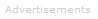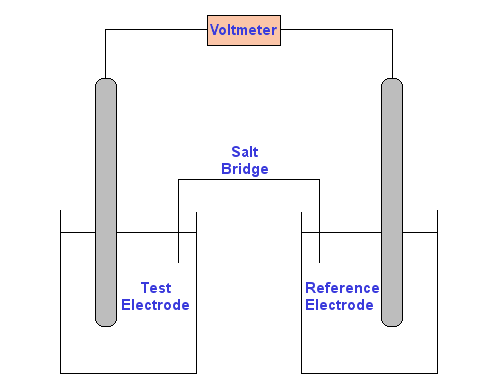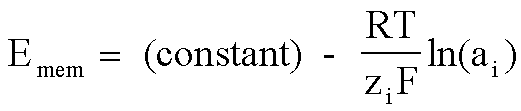# Definition of Potentiometry

What is Potentiometry?

Potentiometry is one of the methods of electroanalytical chemistry. It is usually employed to find the concentration of a solute in solution.

In potentiometric measurements, the potential between two electrodes is measured using a high impedance voltmeter.Use of a high impedance voltmeter in important, because it ensures that current flow is negligible. Since there is no net current, there are no net electrochemical reactions, hence the system is in equilibrium.

At its most fundamental level, a potentiometer consists of two electrodes inserted in two solutions connected by a salt bridge (see diagram below). The voltmeter is attached to the electrodes to measure the potential difference between them.

One of the electrodes is a reference electrode, whose electrode potential is known.

The other electrode is the test electrode.

The test electrode is usually either a metal immersed in a solution of its own ions, whose concentration you wish to discover, or a carbon rod electrode sitting a solution which contains the ions of interest in two different oxidation states.The Nernst Equation can be used to find the concentration of the test solution.

The Nernst equationExample

You wish to find the concentration of Ag+ in a silver nitrate solution. Your reference electrode consists of silver metal in a known 0.1 M silver nitrate solution. Your test electrode is silver metal in the silver nitrate solution you wish to find the concentration of.

Since no current flows, the cell is at equilibrium:

Ag+ ⇌ Ag

The solutions are linked by a 0.1 M potassium nitrate salt bridge. The metal electrodes are linked by a voltmeter.

There will be a potential difference between the two solutions resulting from the different concentrations of silver ions in contact with the silver electrodes. (For example, see J. Chem. Educ., 1995, 72 (8), p A162)

The potential you measure on the voltmeter, Ecell is related to the reference electrode potential and test electrode potential as follows:

Ecell = Ereference - Etest               (Equation 1)

Now, Ereference and Etest can both be expanded using the Nernst Equation:

Ereference = E°Ag/Ag+ - RTlnKeq/nF

Therefore, at a temperature of 298 K:

Ereference = E°Ag/Ag+ - 0.059log10(1/[Ag+reference])               (Equation 2)

Similarly:

Etest = E°Ag/Ag+ - 0.059log10(1/[Ag+test])               (Equation 3)

Now we can substitute Equation 2 and Equation 3 into Equation 1. We can also assume [Ag+reference] is a constant. So, after rearranging, we get:

[Ag+test] = 10-[(Ecell + 0.059)/0.059]               (Equation 4)

The potential reading from the voltmeter is Ecell. All you need to do now is to enter your reading from the voltmeter into Equation 4 and calculate the concentration of silver ions in the test solution.

More

Physical phenomena which do not involve explicit redox reactions, but whose initial conditions have a non-zero free energy, also will generate a potential.

An example of this would be ion concentration gradients across a semi-permeable membrane.

This can also be a potentiometric phenomenon, and is the basis of measurements that use ion-selective electrodes.Instrumentation

See the documents on ion-selective electrodes and pH meters.Search the Dictionary for More Terms